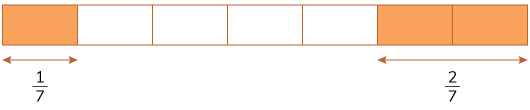# Addition and Subtraction of Fractions

Go back to  'Fractions'

## Addition and Subtraction of Fractions

### Like Fractions

Suppose we are asked to add \begin{align} \frac {1}{7} \end{align} and \begin{align} \frac {2}{7}. \end{align} One way to do this is to represent them both visually.

Let us represent these two fractions on the same strip ($$1$$ unit long).Notice that a total of $$3$$ parts (of $$7$$ parts) of the strip are shaded.
Which means that \begin{align} \frac {1}{7} + \frac {2}{7} = \frac {3}{7} \end{align}

Let us use a strip to subtract \begin{align} \frac {7}{8} \end{align} and \begin{align} \frac {5}{8}. \end{align} We first represent the larger of the two fractions on the strip (in this case \begin{align} \frac {7}{8} \end{align}). We next cross out the smaller fraction on the strip (in this case \begin{align} \frac {5}{8} \end{align}). We then count the number of parts of the shaded region that remain to get to the answer.

Since a total of $$2$$ parts of the shaded region remain,

\begin{align} \frac {7}{8} - \frac {5}{8} = \frac {2}{8}\end{align}

### Unlike Fractions

When you are given two mixed fractions, you just go ahead and add/subtract them as you would two proper fractions. You take the LCM of the two denominators, and make that LCM the denominator of the solution, and multiply the denominators accordingly.

Example:  \begin{align} \frac {2}{5} + \frac {3}{7} = ? \end{align}

\begin{align} \frac {2}{5} = \frac {2 \times 7}{5 \times 7} = \frac {14}{35}\end{align}  and  \begin{align} \frac {3}{7} = \frac {3 \times 5}{7 \times 5} = \frac {15}{35}\end{align}

\begin{align} \frac {14}{35} + \frac {15}{35} = \frac {29}{35}\end{align}

Example:  \begin{align} \frac {41}{8} - \frac {5}{4} = ? \end{align}

\begin{align} \frac {41}{8} = \frac {41 \times 1}{8 \times 1} = \frac {41}{8} \end{align}  and  \begin{align} \frac {5}{4} = \frac {5 \times 2}{4 \times 2} = \frac {10}{8} \end{align}

\begin{align} \frac {41}{8} - \frac {10}{8} = \frac {31}{8} \end{align}

You can also convert your answer to a mixed fraction. So, your final answer will be \begin{align} 3\frac {7}{8}. \end{align}

## Tips and Tricks

• When adding or subtracting unlike fractions, it is not necessary to find the LCM of the denominators. Any common multiple will do. So, simply multiplying the two denominators gives us a common multiple. This may lead to larger looking numbers but is often a quicker way to add or subtract.
E.g. \begin{align} \frac {2}{9} + \frac {1}{12}. \end{align} To do this, first, the LCM of $$9$$ and $$12$$ need to be found. Instead just multiply the first fraction with $$12$$ and second with $$9$$ to get \begin{align} \frac {24}{108} + \frac {9}{108} = \frac {33}{108} = \frac {11}{36}. \end{align}

### Common mistakes or misconceptions

• Children often tend to add the numerators and denominators separately while adding two fractions.
This happens because they see a fraction as two whole numbers instead of one number. So, they perform operations just the way they do with whole numbers. \begin{align} \frac {1}{2} + \frac {3}{4} = \frac {(1 + 3)}{(2 + 4)} = \frac {4}{6} \end{align} because it is addition of a bunch of whole numbers.
• Children subtract the numerators and denominators separately while subtracting two fractions.
This happens because they see a fraction as two whole numbers and perform operations just the way they do with whole numbers. So for them \begin{align} \frac {3}{4} - \frac {1}{2} = \frac {(3 - 1)}{(4 - 2)} = \frac {2}{2} \end{align} because it is subtraction of a bunch of whole numbers.
• When adding or subtracting unlike fractions, the first step is to find equivalent fractions that will form a like pair. To do this, students are expected to find the LCM of the denominators. If the reason behind this step is not clear, students may make various mistakes including taking LCM of the numerator or HCF of the denominator and get stuck.

## Solve the given fractions

a.  \begin{align} \frac {1}{6} + \frac {2}{5} + \frac {5}{8} \end{align}
b.  \begin{align} \frac {9}{2} + \frac {10}{3} - \frac {3}{4} \end{align}
c.  \begin{align} 2\frac {1}{3} + 2\frac {1}{5} - 2\frac {1}{2} \end{align}​​​​​​